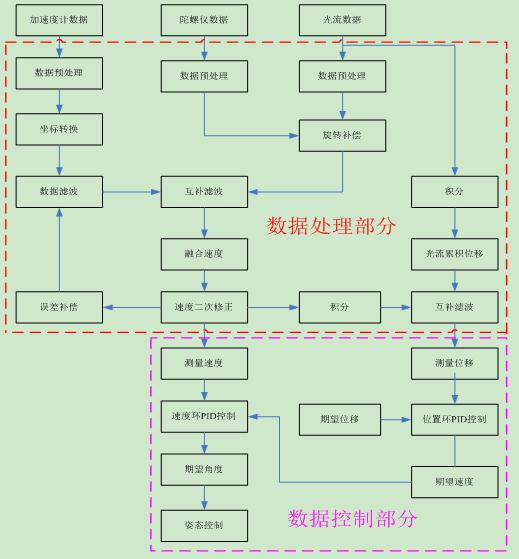# 如何使用光流芯片U30实现四轴无人机悬停

U30输出原始的光流数据并不是直接输出给PID使用，这些数据需要进行加工处理，才能供飞控端使用。对U30输出数据的处理分两种情况：一种是只使用纯光流数据，经过滤波处理得到飞机的位移与速度信息；二是将光流数据与加速度计进行融合，以得到飞控端PID控制所需要的位移和速度信息。

1.只使用光流纯数据的处理方法

1.1 速度计算方法：
speed_x = (h*flow_dat.x/ (integration_timespan*0.000001))*100
speed_y = (h*flow_dat.y/(integration_timespan*0.000001))*100

1.2 位移计算方法：
sum_flow_x += speed_x*(integration_timespan*0.000001);
sum_flow_y += speed_y*(integration_timespan*0.000001);1.3 备注：
1.3.1 由于计算的速度帧率不是很高，且帧间的速度信息可能差别比较大，因此可以根据控制需要，选择对计算的速度进行低通滤波，但不宜引入过大的延时。
1.3.2 理论上在速度计算之前需要进行旋转补偿，但在初始测试时，可以暂时先不考虑，先跑通整个框架。如果悬停效果可以接受也不用进行旋转补偿；若悬停时低频震荡且PID参数怎么也调试不好，就可能需要进行补偿，具体的旋转补偿方法在2.2节中再做详细叙述。
1.3.3 光流位移量的处理有些细节需要注意，在某些情况下需要清除该累积位移与控制积分量。情况一，打摇杆后需要清零，否则松摇杆后飞机还会往前进的反方向后退一段距离；情况二，起飞前或正在起飞时需要清零，否则飞机会斜着起飞，即起飞上升时飞机往一边严重漂移。情况三，悬停时，当累积距离大于一定范围。
1.3.4 在只使用气压计进行定高而没有超声波时，由于气压计会有很大漂移，因此输出的高度要做限制。首先限制高度要非负，另外在较低高度时可以使用固定高度，我们在测试时一般将高度写死固定为1m，实际测试发现在实际高度5m以下，使用该写死的高度也能有较好的悬停效果；当实际高度比较高时，可以适当放大该固定高度比例。
2.光流与加速度计融合的处理方法

fx_gyro_fix = ((fx + LIMIT(((gyro_lpf_y)/57.3f),-flow_t1,flow_t1)) *10 *use_height ) ; //rotation compensation
fy_gyro_fix = ((fy - LIMIT(((gyro_lpf_x)/57.3f),-flow_t2,flow_t2)) *10 *use_height ) ;

(gyro_lpf_y)/57.3为陀螺仪输出角速度，单位rad/s，flow_t1为对陀螺仪输出的限幅，值的大小由fx的输出最大值决定，因为陀螺仪的输出是远远大于光流的输出范围的，限幅避免过度补偿。gyro_lpf_y为原始的陀螺仪角速度(度/秒)，经低通滤波后所得：LPF_1_(3.0f,dT,gyro_x,gyro_lpf_x)，目的是使得光流输出的相位与陀螺仪相位一致。

2.1 对于位置环，期望位置为零，反馈值为光流测量位移，输出为期望速度。
2.2 对于速度环，期望值为位置环的输出，反馈值为光流测量速度，速度环输出为期望角度或期望加速度。理论上，输出应为期望加速度，并通过非线性转换为期望角度，从而传递给姿态环。在demo中我们的输出直接是期望角度，这是因为在小角度时，我们认为期望加速度与角度是线性相关的。
2.3 定点控制中比较重要的一点，要确保外环的执行频率要低于内环的执行频率，否则会造成系统的不稳定。比如，我们位置环的控制周期为40ms,则速度环的控制周期为20ms等等。
2.4 控制量在某些情况下需要清零，特别是控制积分量。比如打摇杆、起飞时或一键翻滚时。

3.1 光流模块的检查。拿到光流芯片后，首先需要检查镜头表面是否有塑料薄膜、水珠、划痕等，确保镜头表面干净，器件或连线无松动等影响镜头成像效果的问题。
3.2 光流模块的安装。在飞机上安装光流模块时，尽量保证镜头的水平，不要有倾斜角度，光流模块与机体坐标系不要有偏航方向的夹角且在机架的旋转中心，即光流模块的轴与机体坐标系的轴应尽量保持重合。特别注意一旦调试通过，进入批量生产时，在其他飞机上都需要以该方向固定安装，不可随意改变安装方向。
3.3初始调试时，接上光流后，使用上位机观察是否有输出，验证输出值是否与图2.所述的光流坐标系定义一致，比如往左水平运动光流Y是否为正；水平向右运动光流Y是否为负，且输出值是否在合理范围等。
3.4 确保光流的输出与机体控制坐标系的一致性，即实飞测试前需要验证光流的控制输出值与期望给飞机的控制指令是否一致(手拿着飞机水平移动，看输出是否符合预期)，避免造成控制指令的不匹配导致的飞机乱飞现象。
3.5 初始调试时应尽量选择光照明亮，地面纹理丰富且不反光，无风的环境进行，避免引入环境的干扰因素。

// up_flow.h

#ifndef _up_flow_H
#define _up_flow_H

#include "stm32f4xx.h"
#include "ms5611.h"

void Flow_Init(void);
void Flow_Duty(float dT);
void Flow_Get(u8);

extern struct flow_float flow_dat;

extern float exp_rol_flow,exp_pit_flow;

struct flow_integral_frame {
unsigned short frame_count_since_last_readout;
signed short pixel_flow_x_integral;
signed short pixel_flow_y_integral;
signed short gyro_x_rate_integral;
signed short gyro_y_rate_integral;
signed short gyro_z_rate_integral;
unsigned int integration_timespan;
unsigned int sonar_timestamp;
unsigned short ground_distance;
signed short gyro_temperature;
unsigned char qual;
} ;

struct flow_float{
float x;
float y;
unsigned short dt;
unsigned char qual;
unsigned char update;
};#endif

// up_flow.c

#define LIMIT( x,min,max ) ( ((x) < (min)) ? (min) : ( ((x) > (max))? (max) : (x) ) )
#define LPF_1_(hz,t,in,out) ((out) += ( 1 / ( 1 + 1 / ( (hz) *3.14f *(t) ) ) ) *( (in) - (out) )) //一阶低通滤波
#define safe_div(numerator,denominator,safe_value) ( (denominator == 0)? (safe_value) : ((numerator)/(denominator)) )

void filter_1(float base_hz,float gain_hz,float dT,float in,_filter_1_st *f1) //动态调整滤波截止频率的一阶滤波
{
LPF_1_(gain_hz,dT,(in - f1->out),f1->a); //低通后的变化量

f1->b = my_pow(in - f1->out); //求一个数平方函数

f1->e_nr = LIMIT(safe_div(my_pow(f1->a),((f1->b) + my_pow(f1->a)),0),0,1); //变化量的有效率，LIMIT 将该数限制在0-1之间，safe_div为安全除法

LPF_1_(base_hz *f1->e_nr,dT,in,f1->out); //低通跟踪
}

_xyz_f_st heading_coordinate_acc;

_xyz_f_st heading_coordinate_speed_fus;
_xyz_f_st heading_coordinate_speed_err_i;

float fx_gyro_fix,fy_gyro_fix;
float fx_o,fy_o;
float f_out_x,f_out_y;
float gyro_lpf_x,gyro_lpf_y;

_filter_1_st f1_fx;
_filter_1_st f1_fy;

float f1_b,f1_g;

//flow_dat.qual：x，y方向光流都有效：255，x有效：2，y有效：1，都无效：0；

float gyro_x,gyro_y;

void flow_fusion(float dT,float fx,float fy,s32 flow_height,u8 pos_hold)
{
float flow_t1 = 1.0,flow_t2 = 1.0;

fx_o = fx *10 *flow_height; //fx，fy（rad/s）-->flow speed (mm/s)
fy_o = fy *10 *flow_height;
u32 use_height=100;

if(flow_height<200) //input height (cm/s)
{
use_height = 100;
}
else if(flow_height<300)
{
use_height = 150;
}
else if(flow_height<400)
{
use_height = 200;
}
else if(flow_height<500)
{
use_height = 250;
}
else if(flow_height<600)
{
use_height = 300;
}
else if(flow_height<1000)
{
use_height = 350;
}
else
{
use_height = 400;
}

if( pos_hold == 1) //in pose hold mode
{

gyro_y = (((s16)sensor.Gyro_deg.y/2 )*2 ); //degree per second
gyro_x = (((s16)sensor.Gyro_deg.x/2 )*2 );

LPF_1_(3.0f,dT,gyro_y,gyro_lpf_y); //gyro low pass filter (delay) for fitting flow data()
LPF_1_(3.0f,dT,gyro_x,gyro_lpf_x);

fx_gyro_fix = ((fx + LIMIT(((gyro_lpf_y)/57.3f),-flow_t1,flow_t1)) *10 *use_height ) ; //rotation compensation
fy_gyro_fix = ((fy - LIMIT(((gyro_lpf_x)/57.3f),-flow_t2,flow_t2)) *10 *use_height ) ;

vec_3d_transition(&imu_data.z_vec, &imu_data.a_acc, &heading_coordinate_acc); //机体坐标系下加速度到水平机体航向坐标系(将机体水平放置，没有偏航角，
//保持加速度与光流没有偏航方向的影响)

f1_fx.out += ((s32)heading_coordinate_acc.x/10 *10 ) *dT; //integrated acceleration for speed
f1_fy.out += ((s32)heading_coordinate_acc.y/10 *10 ) *dT;

f1_g = 2.5f;
if(flow_dat.qual <3) //光流效果不好时
{
f1_b = 0.5f;
}
else
{
if(f1_b<1.2f)
{
f1_b += 0.02f;
}
}

filter_1(f1_b,f1_g,dT,fx_gyro_fix,&f1_fx); //flow_data with acc integrated data complementary filtering
filter_1(f1_b,f1_g,dT,fy_gyro_fix,&f1_fy);

heading_coordinate_speed_fus.x = f1_fx.out; //融合速度
heading_coordinate_speed_fus.y = f1_fy.out;

f_out_x = heading_coordinate_speed_fus.x + 0.1f *heading_coordinate_speed_err_i.x; //融合速度二次修正，最终输出结果
f_out_y = heading_coordinate_speed_fus.y + 0.1f *heading_coordinate_speed_err_i.y;

heading_coordinate_speed_err_i.x += (fx_gyro_fix - f_out_x) *dT;
heading_coordinate_speed_err_i.y += (fy_gyro_fix - f_out_y) *dT;

}

}

...全文
31 点赞 收藏 回复1867

1018